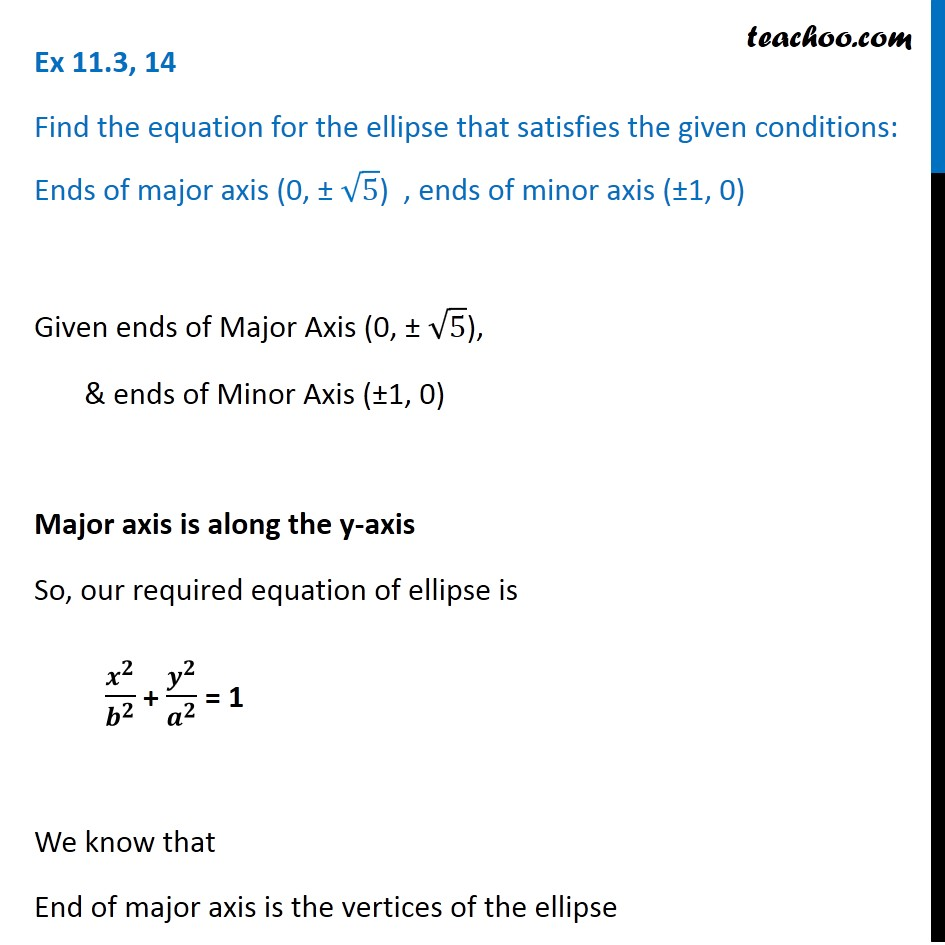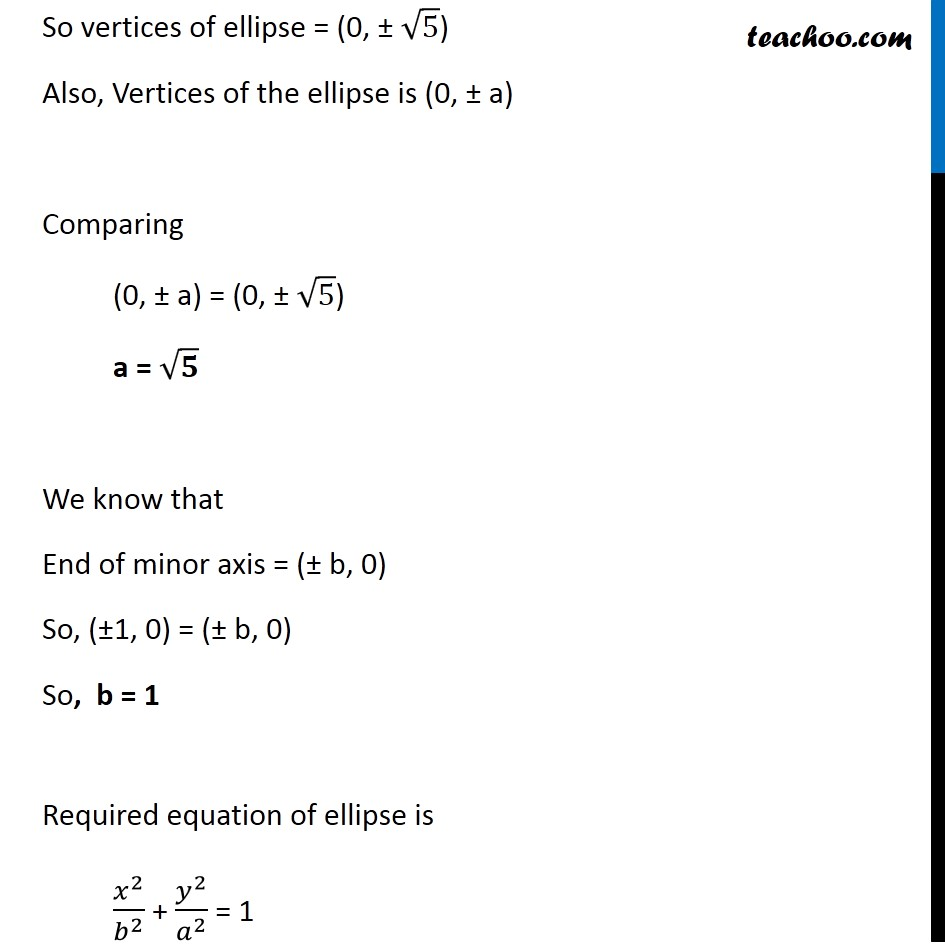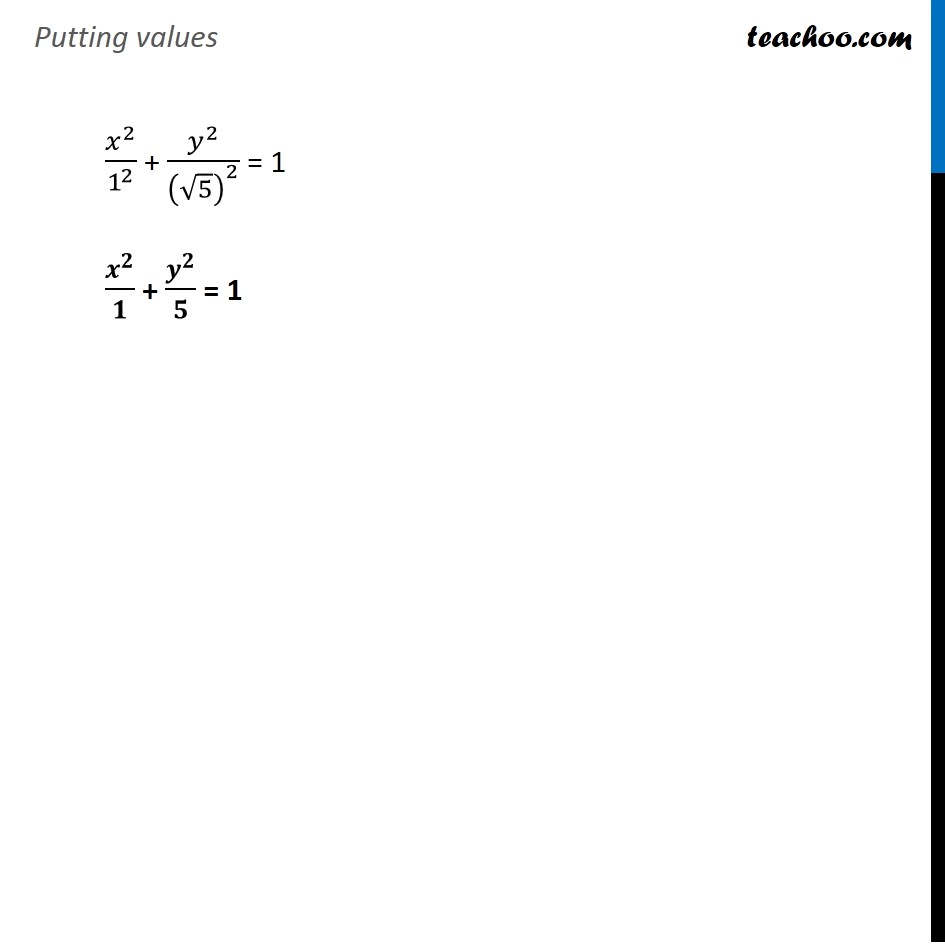Ex 11.3

Chapter 11 Class 11 Conic Sections
Serial order wiseGet live Maths 1-on-1 Classs - Class 6 to 12

### Transcript

Ex 11.3, 14 Find the equation for the ellipse that satisfies the given conditions: Ends of major axis (0, ± √5) , ends of minor axis (±1, 0) Given ends of Major Axis (0, ± √5), & ends of Minor Axis (±1, 0) Major axis is along the y-axis So, our required equation of ellipse is 𝒙^𝟐/𝒃^𝟐 + 𝒚^𝟐/𝒂^𝟐 = 1 We know that End of major axis is the vertices of the ellipse So vertices of ellipse = (0, ± √5) Also, Vertices of the ellipse is (0, ± a) Comparing (0, ± a) = (0, ± √5) a = √𝟓 We know that End of minor axis = (± b, 0) So, (±1, 0) = (± b, 0) So, b = 1 Required equation of ellipse is 𝑥^2/𝑏^2 + 𝑦^2/𝑎^2 = 1 Putting values 𝑥^2/1^2 + 𝑦^2/(√5)^2 = 1 𝒙^𝟐/𝟏 + 𝒚^𝟐/𝟓 = 1